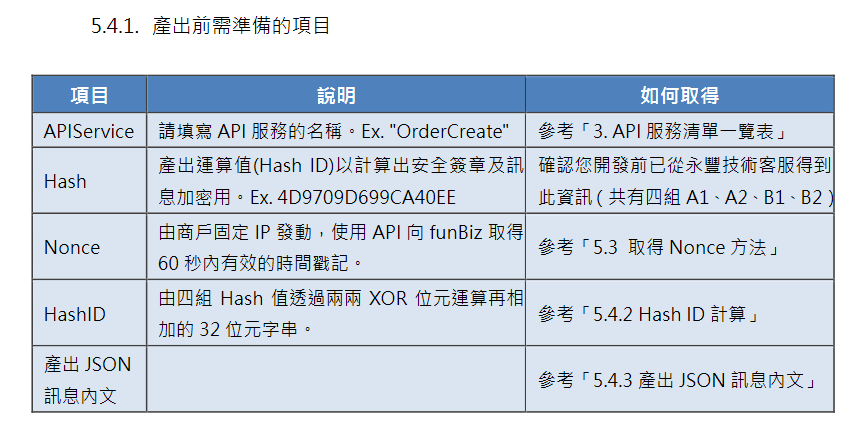#DAY 2
1

## 計算API所需要的參數: Hash IDHash ID是透過位元運算(XOR)將四組Hash計算產出的，將A1/A2以XOR運算所得的字串，再與B1/B2以XOR運算出來的字串，二個相加後將英文轉換為大寫，為長度為32的字串(例: 17D8E6558DC60E702A6B57E1B9B7060D)。

``````
def strToHexByte(s):
return int(s,base=16)

A1 = "4D9709D699CA40EE"
A2 = "5A4FEF83140C4E9E"
B1 = "BC74301945134CB4"
B2 = "961F67F8FCA44AB9"

# 透過 ^ 進行 XOR 計算
XOR1 = strToHexByte(A1) ^ strToHexByte(A2)
XOR2 = strToHexByte(B1) ^ strToHexByte(B2)

# 將 XOR 的結果(數字) 轉成 hex
hex_XOR1 = hex(XOR1)
hex_XOR2 = hex(XOR2)

# 將字串合併後，再大寫
hash_id = ( hex_XOR1 + hex_XOR2 ).upper()
``````

"0X17D8E6558DC60E700X2A6B57E1B9B7060D"

"17D8E6558DC60E702A6B57E1B9B7060D"

``````# 透過 Python 的  字串[開始位置:結束位置]，
# 因為我們不要前面2個符號，所以用 [2:]的方式來截斷文字

hash_id = ( hex_XOR1[2:] + hex_XOR2[2:] ).upper()

``````